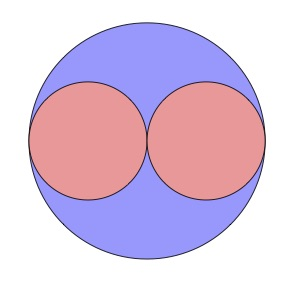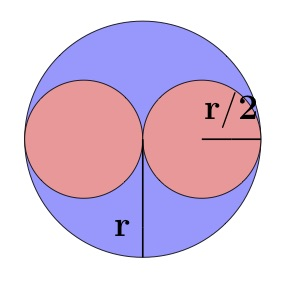# Circles inside Circles

## Problem

Two small circles, shown in pink, are drawn inside a large circle, shown in blue. The two pink circles just fit inside the blue circle. What is the ratio of the area that is shaded blue to the area that is shaded pink?small circles inside big circle

## Solution

Since dimensions aren’t given, it seems the answer may be the same for any size large circle, as long as the small ones are the same as each other and just fit inside the large one. Let’s try that assumption and see if it works.

We’ll need letters to represent the lengths. Let’s have represent the radius of the large circle. Then is the radius of each of the small circles.Two small circles just fit within a larger circle.

The blue area is equal to the area of the large circle minus the combined area of the two small circles. What are those areas?

A circle’s area is

The large circle: The radius is so the circle’s area is

The small circles: The circles’ radius is so the pink area, the combined area of the two small circles, is

Then the blue area, which is the difference between the area of the large circle and the combined areas of the two small circles, is

The blue and pink areas are both multiples of That’s enough for us to find their ratio, so our assumption that we can solve the problem without a number for the radius is validated.

And the ratio of the blue area to the pink area is

0 replies